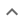•# Simplification or other simple results

We think you wrote:

###This solution deals with simplification or other simple results.

Solution foundx^2+x-4

## Step by Step Solution### Reformatting the input :

(1): "x2"   was replaced by   "x^2".

## Step  1  :

#### Trying to factor by splitting the middle term

Factoring  x2+x-4

The first term is,  x2  its coefficient is  1 .
The middle term is,  +x  its coefficient is  1 .
The last term, "the constant", is  -4

Step-1 : Multiply the coefficient of the first term by the constant   1 • -4 = -4

Step-2 : Find two factors of  -4  whose sum equals the coefficient of the middle term, which is   1 .

 -4 + 1 = -3 -2 + 2 = 0 -1 + 4 = 3

Observation : No two such factors can be found !!
Conclusion : Trinomial can not be factored

## Final result :

  x2 + x - 4